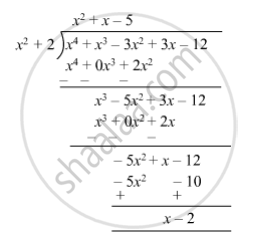SSC (English Medium) Class 8Maharashtra State Board
Share

# Divide and Write the Quotient and the Remainder. (3x − 3x2 − 12 + X4 + X3) ÷ (2 + X2) - SSC (English Medium) Class 8 - Mathematics

ConceptTo Divide a Polynomial by a Binomial

#### Question

Divide and write the quotient and the remainder.

(3x − 3x2 − 12 + x4 + x3) ÷ (2 + x2)

#### Solution

(3x − 3x2 − 12 + x4 + x3) ÷ (2 + x2)So, quotient = $x^2 + x - 5$ and remainder = x $-$ 2.

Is there an error in this question or solution?

#### APPEARS IN

Balbharati Solution for Balbharati Class 8 Mathematics (2019 to Current)
Chapter 10: Division of Polynomials
Practice Set 10.2 | Q: 5 | Page no. 66
Solution Divide and Write the Quotient and the Remainder. (3x − 3x2 − 12 + X4 + X3) ÷ (2 + X2) Concept: To Divide a Polynomial by a Binomial.
S